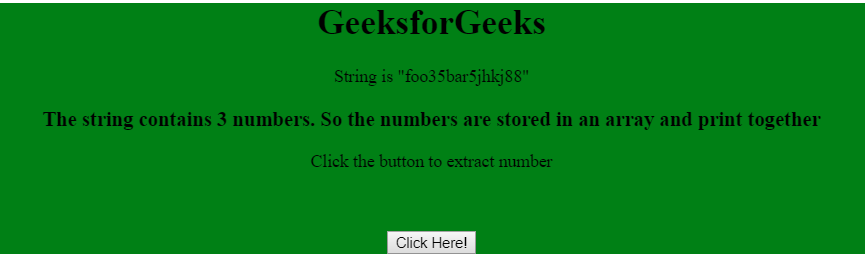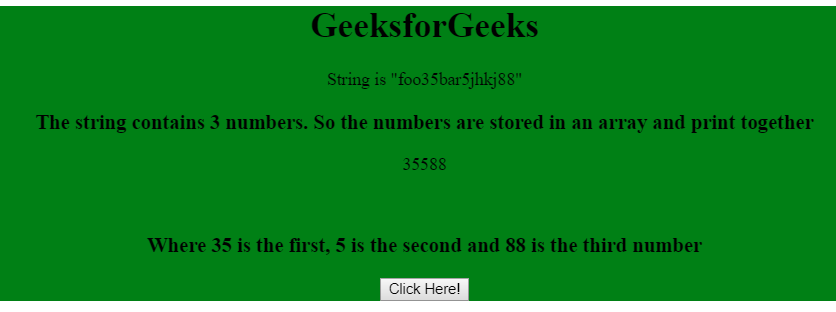# Extract a number from a string using JavaScript

The number from a string in javascript can be extracted into an array of numbers by using the match method. This function takes a regular expression as an argument and extracts the number from the string. Regular expression for extracting a number is (/(\d+)/).

Example 1: This example uses match() function to extract number from string.

 ` ` `<``html``> ` `<``head``> ` `    ``<``title``> ` `        ``Extract number from string ` `    `` ` ` ` ` `  `<``body` `> ` `    ``<``div` `align``=``"center"` `style``=``"background-color: green;"``> ` `         `  `        ``<``h1``>GeeksforGeeks ` `         `  `        ``<``p``>String is "jhkj7682834" ` `         `  `        ``<``p` `id``=``"GFG"``>  ` `            ``Click the button to extract number ` `        `` ` `         `  `        ``<``input` `type``=``"button"` `value``=``"click "` `onclick``=``"myGeeks()"``> ` `    `` ` `     `  `    ``<``script``> ` `        ``function myGeeks() { ` `            ``var str = "jhkj7682834"; ` `            ``var matches = str.match(/(\d+)/); ` `             `  `            ``if (matches) { ` `                ``document.getElementById('GFG').innerHTML ` `                        ``= matches; ` `            ``} ` `        ``} ` `    `` ` ` ` `                                 `

Output:

• Before Clicking the button:• After Clicking the button:Example 2: This example uses match() function to extract number from string.

 ` ` `<``html``> ` `<``head``> ` `    ``<``title``> ` `        ``Extract number from string ` `    `` ` ` ` ` `  `<``body``> ` `    ``<``div` `align``=``"center"` `style``=``"background-color: green;"``> ` `         `  `        ``<``h1``>GeeksforGeeks ` `         `  `        ``<``p``>String is "foo35bar5jhkj88" ` `         `  `        ``<``h3``> ` `            ``The string contains 3 numbers. So the numbers ` `            ``are stored in an array and print together ` `        `` ` `         `  `        ``<``p` `id``=``"GFG"``> ` `            ``Click the button to extract number ` `        ``<``br``> ` `         `  `        ``<``h3` `id``=``"Geeks"``> ` `         `  `        ``<``input` `type``=``"button"` `value``=``"Click Here!"` `onclick``=``"myGeeks()"``> ` `    `` ` `     `  `    ``<``script``> ` `        ``function myGeeks() { ` `            ``var str = "foo35bar5jhkj88"; ` `            ``matches = str.match(/\d+/g); ` `            ``var i=0 ` `         `  `            ``document.getElementById('GFG').innerHTML ` `                ``= matches + matches + matches; ` `             `  `            ``document.getElementById("Geeks").innerHTML ` `                ``= "Where 35 is the first, 5 is the second" ` `                ``+ " and 88 is the third number" ` `    ``} ` `    `` ` ` ` `                     `

Output:

• Before Clicking the button:• After Clicking the button:My Personal Notes arrow_drop_upCheck out this Author's contributed articles.

If you like GeeksforGeeks and would like to contribute, you can also write an article using contribute.geeksforgeeks.org or mail your article to contribute@geeksforgeeks.org. See your article appearing on the GeeksforGeeks main page and help other Geeks.

Please Improve this article if you find anything incorrect by clicking on the "Improve Article" button below.